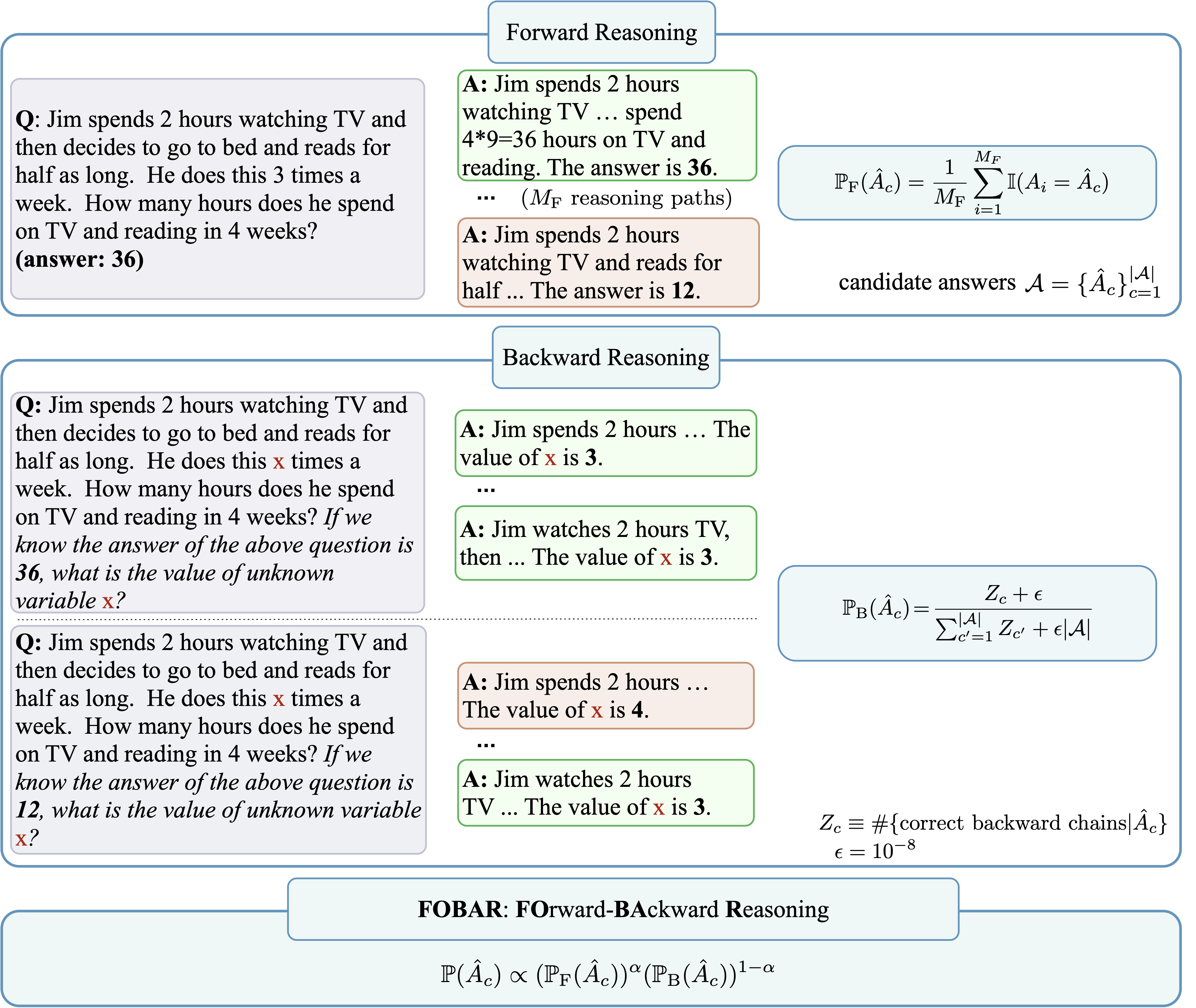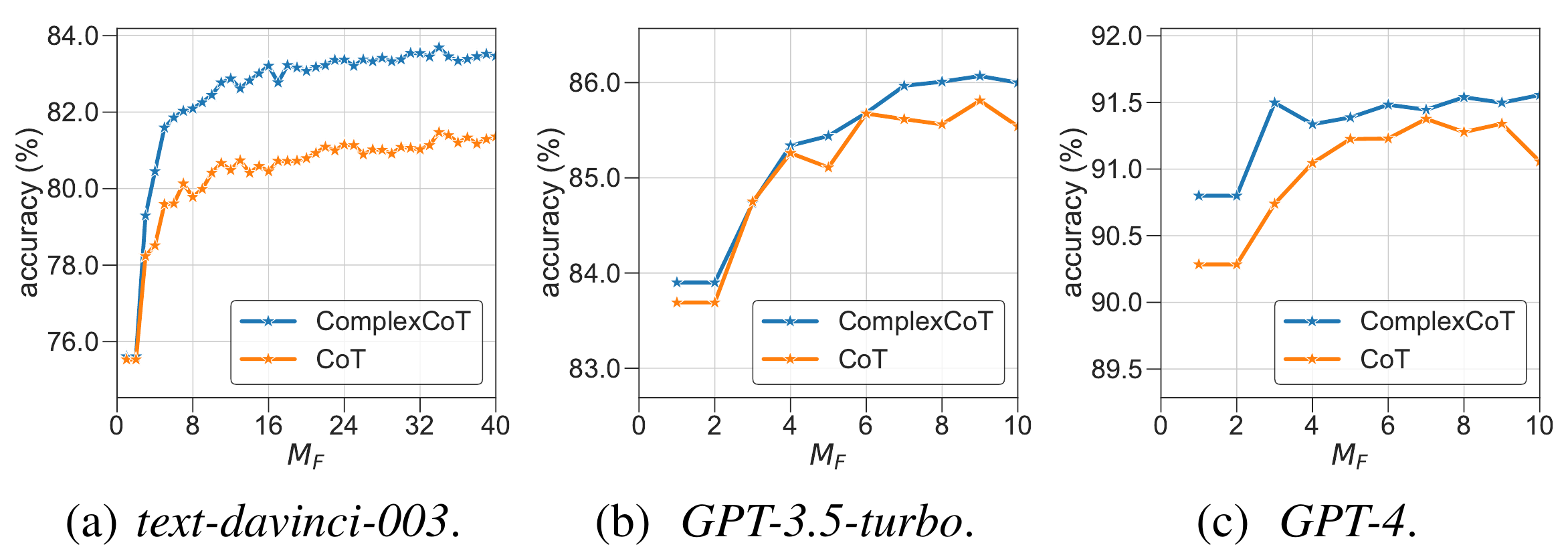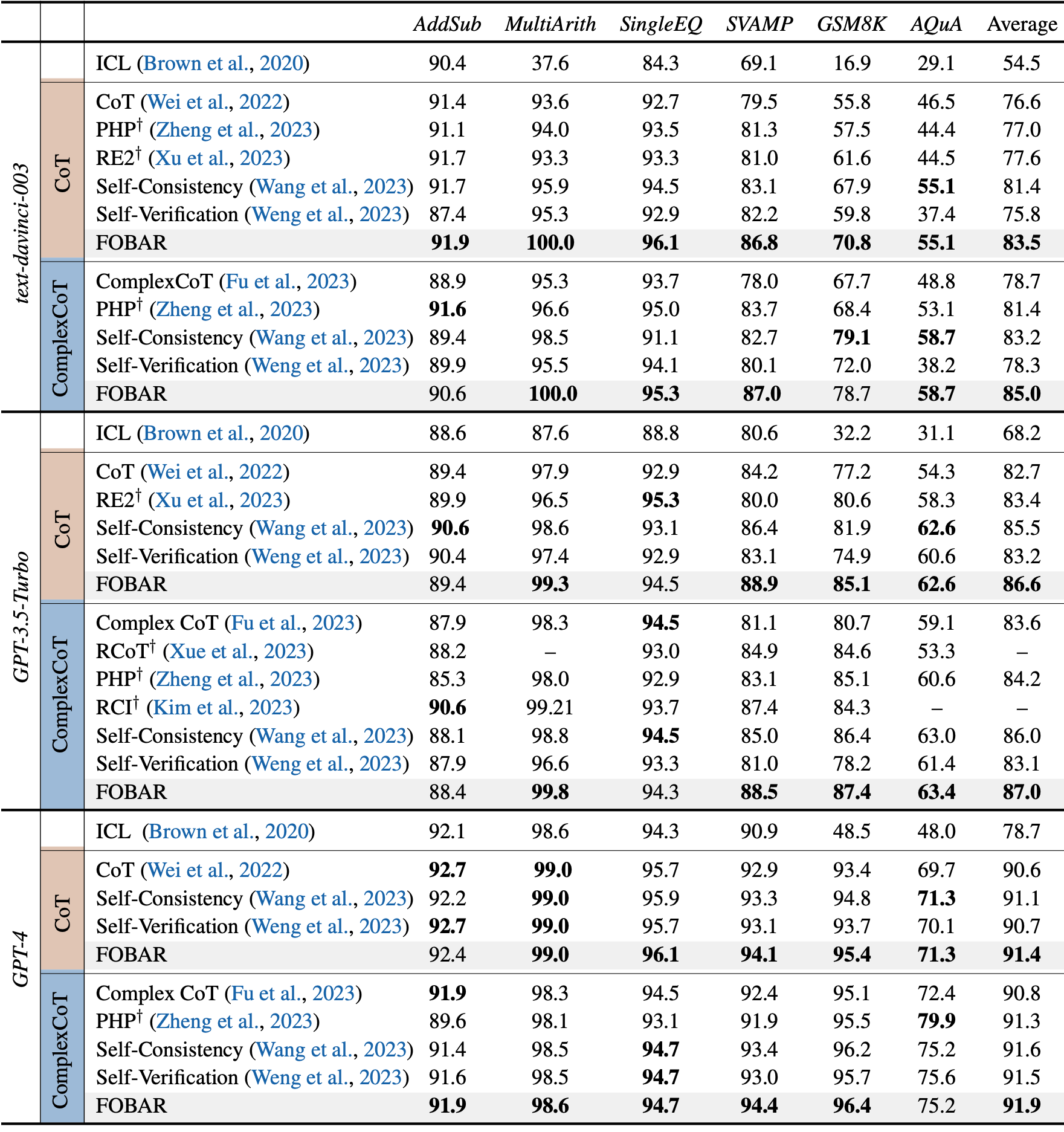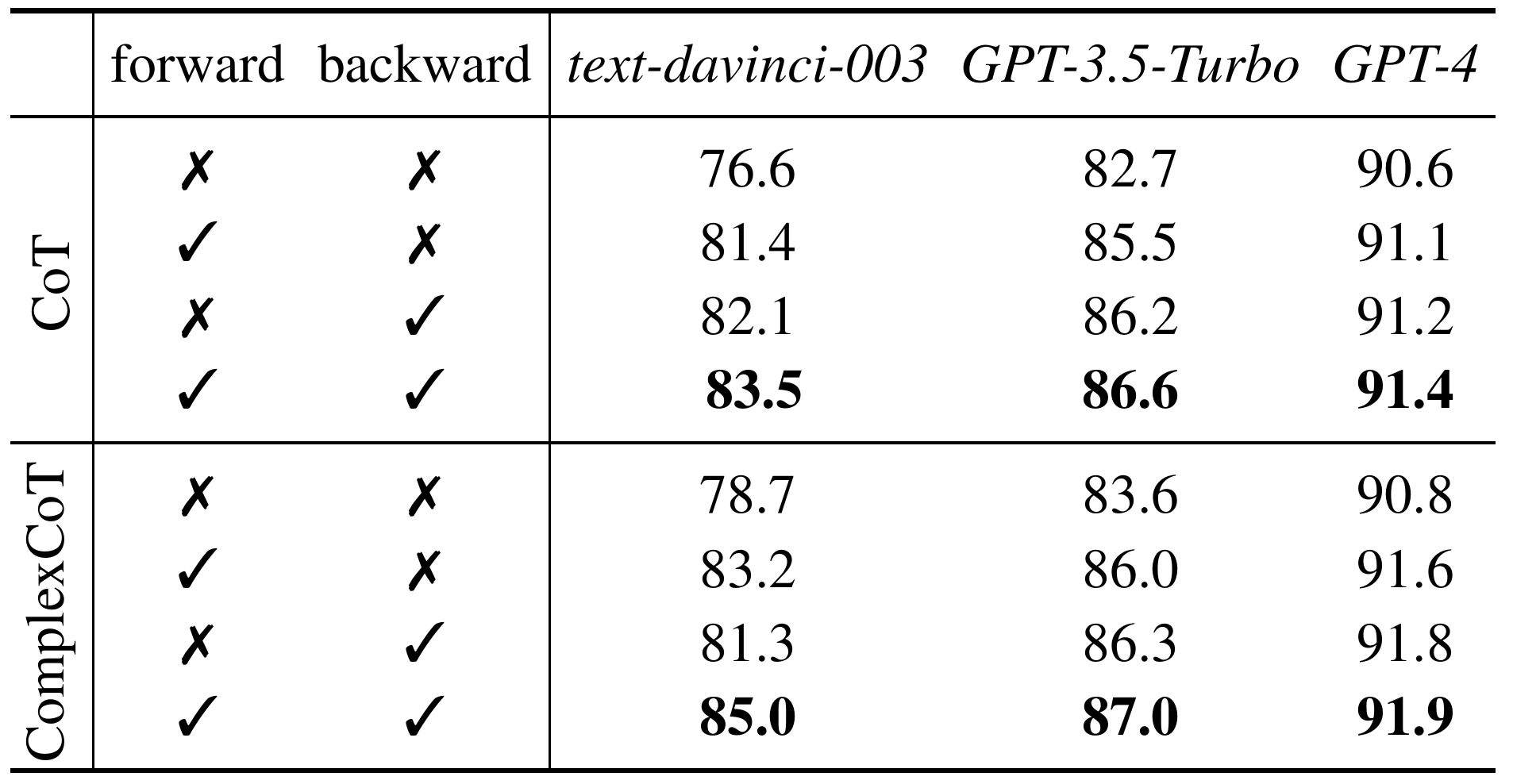# Forward-Backward Reasoning in Large Language Models for Mathematical Verification

1Southern University of Science and Technology, 2Hong Kong University of Science and Technology
3Huawei Noah’s Ark Lab, 4Peking University, 5Peng Cheng Laboratory## Abstract

Chain-of-Thought (CoT) prompting in large language models (LLMs) has shown promising performance on mathematical reasoning tasks. Recently, Self-Consistency samples a diverse set of reasoning chains with different answers and chooses the answer by majority voting. Though effective, its performance cannot be further improved by sampling more reasoning chains. To address this problem, we propose to integrate backward reasoning into answer verification. We first mask a number in the question by ${\bf x}$. The LLM is then asked to predict the masked number with a candidate answer $\hat{A}_c$ embedded in the template: "If we know the answer to the above question is $\hat{A}_c$, what is the value of unknown variable ${\bf x}$?" The LLM is expected to predict the masked number successfully if the provided candidate answer is correct. To further improve performance, we propose FOBAR (FOrward-BAckward Reasoning) to combine forward and backward reasoning for verifying candidate answers. Experiments are performed on six standard mathematical data sets and three LLMs (text-davinci-003, GPT-3.5-Turbo, GPT-4). Results show that FOBAR achieves state-of-the-art performance. In particular, FOBAR outperforms Self-Consistency which uses forward reasoning alone, demonstrating that combining forward and forward reasoning is better. It also outperforms existing verification methods, verifying the effectiveness of using the simple template in backward reasoning and the proposed combination.

## Contributions

Introduce Backward Reasoning to Mathematical Verification

1. A simple template is proposed to create backward questions: We mask a number in the question, and append the template with a candidate answer
2. We design a CoT prompt for the LLM to predict the masked number
3. We estimate the probability of the candidate answer based on the number of correct chains in the backward direction

Propose FOBAR to Combine Forward and Backward Reasoning for Verifying Candidate Answers

1. Combine forward and backward probabilities of candidate answers in a soft manner $$\mathbb{P} (\hat{A}_c) \propto (\mathbb{P} (\hat{A}_c; \text{forward}))^{\alpha} (\mathbb{P} (\hat{A}_c; \text{backward}))^{1-\alpha}$$
2. When $\alpha=1$, it recovers the SOTA Self-Consistency
3. When $\alpha=0$, it recovers the Backward Reasoning for Verification
4. The combination is flexible, other methods like arithmetic mean can be used

Extensive Experiments on Various Mathematical Data Sets

1. FOBAR achieves SOTA performance
2. FOBAR outperforms Self-Consistency, demonstrating that combining forward and backward reasoning together is better
3. FOBAR achieves higher accuracy than Self-Verification, confirming that using the simple template in backward reasoning and the proposed combination is more effective

### Performance of Self-Consistency Saturates Quickly When Increasing #Paths $M_F$## Template for Generating Questions in Backward Reasoning

Question

Jim spends 2 hours watching TV and then decides to go to bed and reads for half as long. He does this 3 times a week. How many hours does he spend on TV and reading in 4 weeks? (ground-truth answer: 36)

Template

If we know the answer to the above question is $\hat{A}_c$, what is the value of unknown variable ${\bf x}$?

Questions for Backward Reasoning (candidate answer: 36)

Jim spends ${\bf x}$ hours watching TV and then decides to go to bed and reads for half as long. He does this 3 times a week. How many hours does he spend on TV and reading in 4 weeks? If we know the answer to the above question is 36, what is the value of unknown variable ${\bf x}$?

Jim spends 2 hours watching TV and then decides to go to bed and reads for half as long. He does this ${\bf x}$ times a week. How many hours does he spend on TV and reading in 4 weeks? If we know the answer to the above question is 36, what is the value of unknown variable ${\bf x}$?

Jim spends 2 hours watching TV and then decides to go to bed and reads for half as long. He does this 3 times a week. How many hours does he spend on TV and reading in ${\bf x}$ weeks? If we know the answer to the above question is 36, what is the value of unknown variable ${\bf x}$?

Questions for Backward Reasoning (candidate answer: 12)

Jim spends ${\bf x}$ hours watching TV and then decides to go to bed and reads for half as long. He does this 3 times a week. How many hours does he spend on TV and reading in 4 weeks? If we know the answer to the above question is 12, what is the value of unknown variable ${\bf x}$?

Jim spends 2 hours watching TV and then decides to go to bed and reads for half as long. He does this ${\bf x}$ times a week. How many hours does he spend on TV and reading in 4 weeks? If we know the answer to the above question is 12, what is the value of unknown variable ${\bf x}$?

Jim spends 2 hours watching TV and then decides to go to bed and reads for half as long. He does this 3 times a week. How many hours does he spend on TV and reading in ${\bf x}$ weeks? If we know the answer to the above question is 12, what is the value of unknown variable ${\bf x}$?

## Experiments

### Main Results### Usefulness of Forward and Backward Reasoning## BibTeX


@TechReport{jiang2023backward,
title={Forward-Backward Reasoning in Large Language Models for Mathematical Verification},
author={Jiang, Weisen and Shi, Han and Yu, Longhui and Liu, Zhengying and Zhang, Yu and Li, Zhenguo and Kwok, James T},
type={Preprint},
number={arXiv:2308.07758},
year={2023}
}